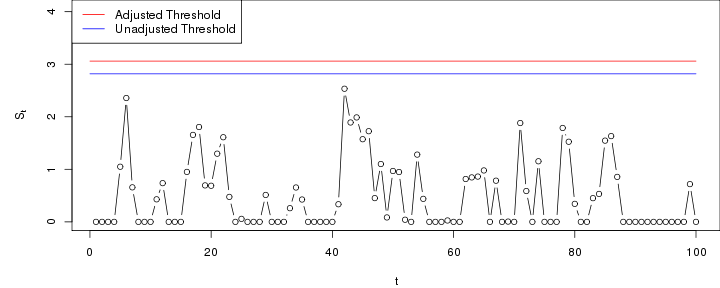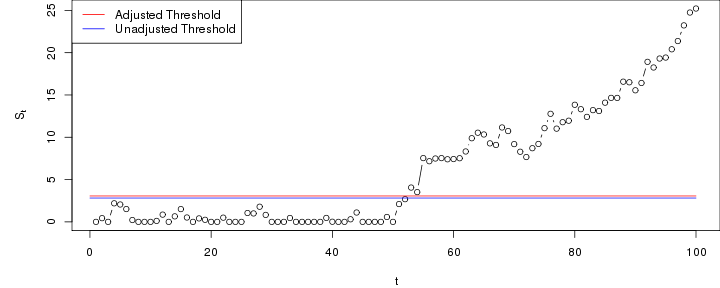# CUSUM chart based on linear regression model

The following is an example of an application to a CUSUM chart based on a linear regression model.

Assume we have $$n$$ past in-control data $$(Y_{-n},X_{-n}),\ldots,(Y_{-1},X_{-1})$$, where $$Y_i$$ is a response variable and $$X_i$$ is a corresponding vector of covariates. The parameters $$\beta$$ of a linear model $$\mbox{E} Y=X\beta$$ are estimated using e.g. the lm function. The corresponding risk adjusted CUSUM chart to detect a shift of $$\Delta>0$$ in the mean of the response for new observations $$(Y_{1},X_{1}),\ldots,(Y_{n},X_{n})$$ is then defined by $S_0=0, \quad S_t=\max\left(0,S_{t-1}+Y_t-X_t\hat\beta-\Delta/2 \right).$

The following generates a data set of past observations (replace this with your observed past data) from the model $$\mbox{E}Y=2+x_1+x_2+x_3$$ with standard normal noise and distribution of the covariate values as specified below.

n <- 1000
Xlinreg <- data.frame(x1= rbinom(n,1,0.4), x2= runif(n,0,1), x3= rnorm(n))
Xlinreg$y <- 2 + Xlinreg$x1 + Xlinreg$x2 + Xlinreg$x3 + rnorm(n)


Next, we initialise the chart and compute the estimate needed for running the chart - in this case $$\hat \beta$$.

library(spcadjust)
chartlinreg <- new("SPCCUSUM",model=SPCModellm(Delta=1,formula="y~x1+x2+x3"))
xihat <- xiofdata(chartlinreg,Xlinreg)
xihat

##
## Call:
## lm(formula = formula, data = P)
##
## Coefficients:
## (Intercept)           x1           x2           x3
##       2.003        1.091        1.003        1.016


## Calibrating the chart to a given average run length (ARL)

We now compute a threshold that with roughly 90% probability results in an average run length of at least 100 in control. In this regression model this is computed by non-parametric bootstrapping. The number of bootstrap replications (the argument nrep) shoud be increased for real applications.

cal <- SPCproperty(data=Xlinreg,
nrep=100,
property="calARL",chart=chartlinreg,params=list(target=100),quiet=TRUE)
cal

## 90 % CI: A threshold of 3.06 gives an in-control ARL of at least
##   100.
## Unadjusted result:  2.817
## Based on  100 bootstrap repetitions.


## Run the chart

Next, we run the chart with new observations that are in-control.

n <- 100
newXlinreg <- data.frame(x1= rbinom(n,1,0.4), x2= runif(n,0,1), x3=rnorm(n))
newXlinreg$y <- 2 + newXlinreg$x1 + newXlinreg$x2 + newXlinreg$x3 + rnorm(n)
S <- runchart(chartlinreg, newdata=newXlinreg,xi=xihat)In the next example, the chart is run with data that that are out-of-control from time 51 and onwards.

n <- 100
newXlinreg <- data.frame(x1= rbinom(n,1,0.4), x2= runif(n,0,1),x3=rnorm(n))
outind <- c(rep(0,50),rep(1,50))
newXlinreg$y <- 2 + newXlinreg$x1 + newXlinreg$x2 + newXlinreg$x3 + rnorm(n)+outind
S <- runchart(chartlinreg, newdata=newXlinreg,xi=xihat)